# RBSE Maths Class 12 Chapter 4: Determinants Important Questions and Solutions

RBSE Class 12 Maths Chapter 4 – Determinants Important questions and solutions are given here. All these important questions and solutions are prepared by our subject experts, and hence the solutions are accurate. The RBSE Class 12 important questions and solutions provided at BYJU’S will help the students in understanding all the concepts of Class 12 in detail.

Chapter 4 of RBSE Class 12 contains two exercises which involve questions on finding the determinant of the given matrix using different methods. These methods of simplification involve the concepts of minors, cofactors, row-wise and column-wise operations on the elements of the matrix. Besides, different properties of the determinant of a matrix are given in this chapter which will help the students for their higher classes.

### RBSE Maths Chapter 4: Exercise 4.1 Textbook Important Questions and Solutions

Question 1: For what value of k is the value of the determinant

$$\begin{array}{l}\begin{vmatrix} k & 2\\ 4 & -3 \end{vmatrix}\end{array}$$
zero?

Solution:

Given,

$$\begin{array}{l}\begin{vmatrix} k & 2\\ 4 & -3 \end{vmatrix}=0\end{array}$$

⇒ k × (-3) – 2 × (4) = 8

⇒ -3k – 8 = 0

⇒ -3k = 8

⇒ k = -8/3

Hence, the value of k is -8/3.

Question 2: If

$$\begin{array}{l}\begin{vmatrix} 2 & 3\\ y & x \end{vmatrix}=4\end{array}$$
and
$$\begin{array}{l}\begin{vmatrix} x & y\\ 4 & 2 \end{vmatrix}=7\end{array}$$
, then find the value of x and y.

Solution:

Given,

$$\begin{array}{l}\begin{vmatrix} 2 & 3\\ y & x \end{vmatrix}=4\end{array}$$

2x – 3y = 4 ….(i)

And,

$$\begin{array}{l}\begin{vmatrix} x & y\\ 4 & 2 \end{vmatrix}=7\end{array}$$

2x – 4y = 7 ….(ii)

Subtracting (i) from (ii),

2x – 4y -(2x – 3y) = 7 – 4

-4y + 3y = 3

-y = 3

⇒ y = -3

Substituting y = -3 in (i),

2x – 3(-3) = 4

2x + 9 = 4

2x = 4 – 9

2x = -5

x = -5/2

Therefore, the value of x = -5/2 and y = -3.

Question 3: Evaluate the determinant and also find the minors and cofactors of elements of the first row.

$$\begin{array}{l}\begin{vmatrix} 1 & -3 & 2\\ 4 & -1 & 2\\ 3 & 5 & 2 \end{vmatrix}\end{array}$$

Solution:

Let

$$\begin{array}{l}A =\begin{vmatrix} 1 & -3 & 2\\ 4 & -1 & 2\\ 3 & 5 & 2 \end{vmatrix}\end{array}$$

Minor of a11:

$$\begin{array}{l}M_{11}=\begin{vmatrix} -1 & 2\\ 5 & 2 \end{vmatrix}\end{array}$$

= -1(2) – 2(5)

= -2 – 10

= -12

Cofactor of a11 = F11 = (-1)2M11

= = 1 x (- 12)

= -12

Minor of a12:

$$\begin{array}{l}M_{12}=\begin{vmatrix} 4 & 2\\ 3 & 2 \end{vmatrix}\end{array}$$

= 4(2) – 2(3)

= 8 – 6

= 2

Cofactor of a12 = F12 = (-1)3M12

= -1 x (2)

= -2

Minor of a13:

$$\begin{array}{l}M_{13}=\begin{vmatrix} 4 & -1\\ 3 & 5 \end{vmatrix}\end{array}$$

= 4(5) – (-1)3

= 20 + 3

= 23

Cofactor of a13 = F13 = (-1)4M13

= 1 x (23)

= 23

Determinant of A = a11F11 + a12F12 + a13F13

= 1(-12) + (-3)(-2) + 2(23)

= -12 + 6 + 46

= 40

Question 4: Prove that: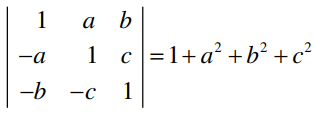Solution:

$$\begin{array}{l}LHS =\begin{vmatrix} 1 & a & b\\ -a & 1 & c\\ -b & -c & 1 \end{vmatrix}\end{array}$$

= 1(1 + c2) -a(-a + bc) + b(ac + b)

= 1 + c2 + a2 – abc + abc + b2

= 1 + a2 + b2 + c2

= RHS

Hence proved.

### RBSE Maths Chapter 4: Exercise 4.2 Textbook Important Questions and Solutions

Question 5: If

$$\begin{array}{l}\begin{vmatrix} l & m\\ 2 & 3 \end{vmatrix}=0\end{array}$$
, then find the ratio l : m.

Solution:

Given,

$$\begin{array}{l}\begin{vmatrix} l & m\\ 2 & 3 \end{vmatrix}=0\end{array}$$

⇒ l x 3 – 2 x m = 0

⇒ 3l – 2m = 0

⇒ 3l = 2m

⇒ l/m = 2/3

Therefore, l : m = 2 : 3.

Question 6: Evaluate the determinant:

$$\begin{array}{l}\begin{vmatrix} 13 & 16 & 19\\ 14 & 17 & 20\\ 15 & 18 & 21 \end{vmatrix}\end{array}$$

Solution: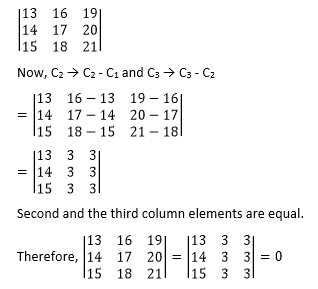Question 7: Solve the following determinant.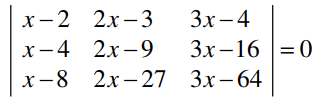Solution:

Given,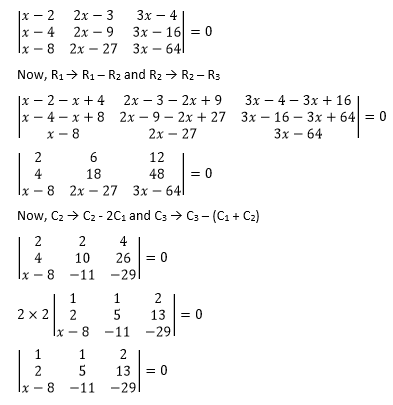⇒ 1(- 145 + 143) – 1(- 58 + 13x + 104) + 2( – 22 – 5x +40) = 0

⇒ – 2 + 13x – 46 – 10x + 36 = 0

⇒ 3x – 12 = 0

⇒ 3x = 12

⇒ x = 12/3

⇒ x = 4

Question 8: Without expanding, evaluate the determinant.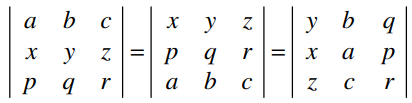Solution: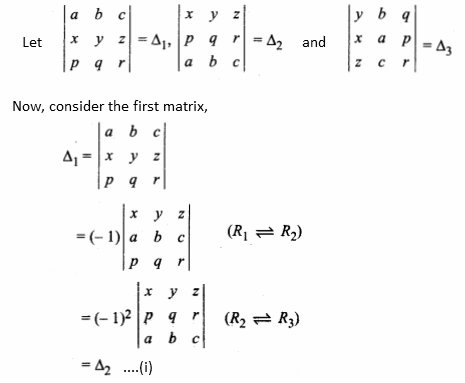Consider the first matrix again,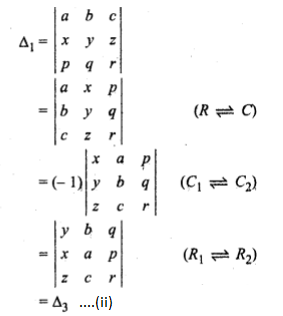From (i) and (ii),

Δ1 = Δ2 = Δ3

Hence proved.

Question 9: If ω is the cube root of unity, then find the value of the determinant

$$\begin{array}{l}\begin{vmatrix} 1& \omega ^3 & \omega^2\\ \omega^3 & 1 & \omega \\ \omega^2 & \omega & 1 \end{vmatrix}\end{array}$$
.

Solution:

We know that, ω3 = 1.

$$\begin{array}{l}\begin{vmatrix} 1& \omega ^3 & \omega^2\\ \omega^3 & 1 & \omega \\ \omega^2 & \omega & 1 \end{vmatrix} = \begin{vmatrix} 1& 1 & \omega^2\\ 1 & 1 & \omega \\ \omega^2 & \omega & 1 \end{vmatrix}\end{array}$$

= 1(1 – ω2) – 1(1 – ω3) + ω2(ω – ω2)

= 1 – ω2 – 1 + ω3 + ω3 – ω4

= 1 – ω2 – 1 + 1 + 1 – ω3.ω (∵ ω3 = 1)

= 3 – ω2 – 1 – ω (∵ ω3 = 1)

= 3 – (1 + ω + ω2)

= 3 – 0 (∵ 1 + ω + ω2 = 0)

= 3

### RBSE Maths Chapter 4: Additional Important Questions and Solutions

Question 1: The value of the determinant

$$\begin{array}{l}\begin{vmatrix} cos80^{\circ} & -cos10^{\circ}\\ sin80^{\circ} & sin10^{\circ} \end{vmatrix}\end{array}$$
is

(a) 0

(b) 1

(c) -1

(d) none of these

Solution:

$$\begin{array}{l}\begin{vmatrix} cos80^{\circ} & -cos10^{\circ}\\ sin80^{\circ} & sin10^{\circ} \end{vmatrix}\end{array}$$

= cos 80° sin 10° – (- cos 10°) sin 80°

= cos 80° sin 10° + cos 10° sin 80°

= sin (10° + 80°)

= sin 90°

= 1

Question 2: The cofactors of first column in the determinant

$$\begin{array}{l}\begin{vmatrix} 5 & 20\\ 3 & -1 \end{vmatrix}\end{array}$$
are

(a) -1, 3

(b) -1, -3

(c) -1, 20

(d) -1, -20

Solution:

Let

$$\begin{array}{l}A= \begin{vmatrix} 5 & 20\\ 3 & -1 \end{vmatrix}\end{array}$$

Cofactor of a11 = F11 = (- 1)2 M11

= 1 x (- 1)

= – 1

Cofactor of a12 = F21 = (-1)3 M21

= (- 1) × 20

= – 20

Hence, the cofactors of the given determinant are -1 and -20.

Question 3: If

$$\begin{array}{l}\Delta =\begin{vmatrix} 1 & 2 & 3\\ 4 & 5 & 6\\ 1 & 2 & 4 \end{vmatrix}\end{array}$$
, then the value of the determinant
$$\begin{array}{l}\begin{vmatrix} -2 & -4 & -6\\ -8 & -10 & -12\\ -2 & -4 & -8 \end{vmatrix}\end{array}$$
is

(a) -2Δ

(b) 8Δ

(c) -8Δ

(d) -6Δ

Solution:

Given,

$$\begin{array}{l}\Delta =\begin{vmatrix} 1 & 2 & 3\\ 4 & 5 & 6\\ 1 & 2 & 4 \end{vmatrix}\end{array}$$

$$\begin{array}{l}\begin{vmatrix} -2 & -4 & -6\\ -8 & -10 & -12\\ -2 & -4 & -8 \end{vmatrix}=(-2)(-2)(-2)\begin{vmatrix} 1 & 2 & 3\\ 4 & 5 & 6\\ 1 & 2 & 4 \end{vmatrix}=-8\Delta\end{array}$$

Question 4: If

$$\begin{array}{l}\begin{vmatrix} 4 & 1\\ 2 & 1 \end{vmatrix}^2=\begin{vmatrix} 3 & 2\\ 1 & x \end{vmatrix}-\begin{vmatrix} x & 3\\ -2 & 1 \end{vmatrix}\end{array}$$
, then the value of x is

(a) 6

(b) 7

(c) 8

(d) 0

Solution:

Given,

$$\begin{array}{l}\begin{vmatrix} 4 & 1\\ 2 & 1 \end{vmatrix}^2=\begin{vmatrix} 3 & 2\\ 1 & x \end{vmatrix}-\begin{vmatrix} x & 3\\ -2 & 1 \end{vmatrix}\end{array}$$

⇒ (4 – 2)2 = (3x – 2) – (x + 6)

⇒ (2)2 = 3x – 2 – x – 6

⇒ 4 = 2x – 8

⇒ 4 + 8 = 2x

⇒ 2x = 12

⇒ x = 12/2

⇒ x = 6

Question 5: Prove that one root of the equation is x = 2 and hence find the remaining roots.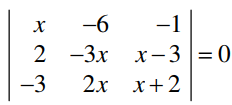Solution: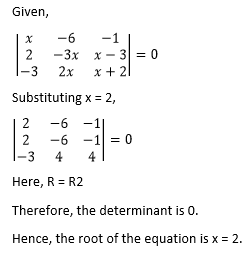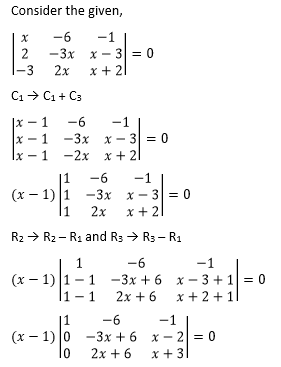⇒ (x – 1)[(-3x + 6)(x + 3) – (2x + 6)(x – 2)] = 0

⇒ (x – 1)[-3(x – 2)(x + 3) – 2(x + 3)(x – 2)] = 0

⇒ -5(x – 1)(x – 2)(x + 3) = 0

⇒ (x – 1)(x – 2)(x + 3) = 0

⇒ x = 1, 2, -3

Therefore, the remaining roots are 1 and -3.